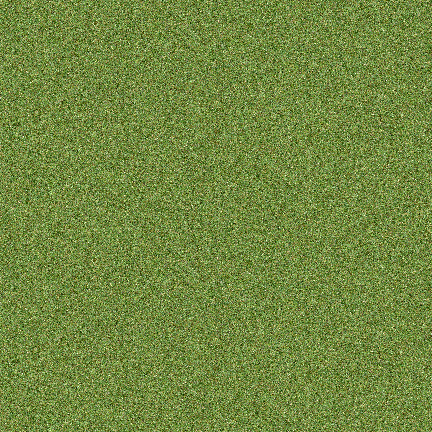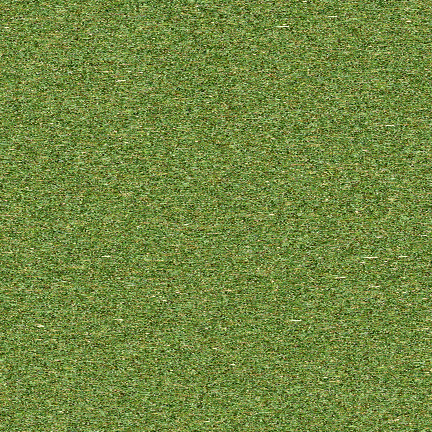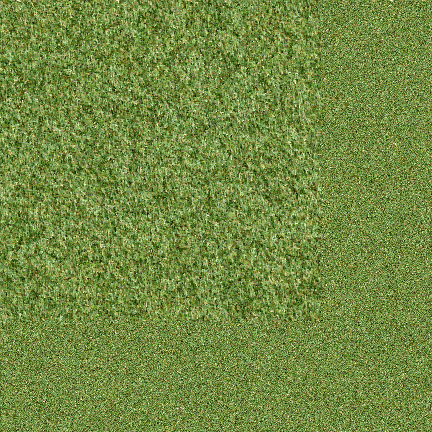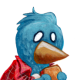# Procedural Texture Generation

Last week I mentioned that I’ve been playing around with procedural texture generation. The method I’ve been using takes an image sample, analyzes it, and then tries to reproduce its texture in several ways. For example, take this picture of grass from the public domain West’s Textures set.The source image shouldn’t have too many colors in it, so before feeding it to the texture generator I edited it in Gimp and posterized it down to around 10 colors.

The first texture generation method is shuffle, which just takes all the pixels from the source image and spits them back out in random order. This is good for generating a noisy texture which has the same color composition as the original, but the output lacks structure.Next, I tried a markov chain, which records left-to-right pixel patterns from the original and tries to replicate these in the output. This one has a bit more structure to it, but the generated texture often appears streaky.Next I tried another markov chain type algorithm, but instead of checking the state of the previous three pixels it examines the pixel above, behind, and to the upper left of the pixel to be drawn.Finally, I tried an extended version of the above algorithm, which looks at more nearby tiles and falls back to a previous algorithm if an unknown seed case is encountered. Sometimes this seems to improve the results and sometimes it doesn’t.You can see the Python script I used below the fold.

```import pygame
import random
import collections

SCREENSIZE = 432

if __name__=='__main__':
import random

# Set the screen size.
screen = pygame.display.set_mode( (SCREENSIZE,SCREENSIZE) )

# Analyze the sample.

# There are three ways to try and copy a terrain texture- the first is just
# to apply random colors with the same frequency as the sample. The second
# is to use a left to right markov chain generator to decide on pixel colors.
# The third way is associating color pixels with the pixel to the left of
# current and to the top of current.
spots = []
mc_spots = collections.defaultdict( list )
lt_spots = collections.defaultdict( list )
xlt_spots = collections.defaultdict( list )

def safe_get_at( sample, x, y ):
try:
c = tuple( sample.get_at( (x,y) ) )
except IndexError:
c = (0,0,0,255)
return c

last_a,last_b,last_c = (0,0,0,255),(0,0,0,255),(0,0,0,255)
left_c,top_c = (0,0,0,255),(0,0,0,255)

for y in range( sample.get_height() ):
for x in range( sample.get_width() ):
c = tuple( sample.get_at( (x,y) ) )
if c != (0,0,0,255):
spots.append( c )
mc_spots[(last_a,last_b,last_c)].append(c)
last_a,last_b,last_c = last_b,last_c,c
l_c = safe_get_at( sample, x-1, y )
t_c = safe_get_at( sample, x, y-1 )
d_c = safe_get_at( sample, x-1, y-1 )
lt_spots[ (l_c,t_c,d_c) ].append( c )

xl_c = safe_get_at( sample, x-2, y )
xlt_spots[ (xl_c,l_c,t_c,d_c) ].append( c )

#spots = spots * 100
random.shuffle( spots )

i = 0
last_a,last_b,last_c = (0,0,0,255),(0,0,0,255),(0,0,0,255)
for y in range( SCREENSIZE ):
for x in range( SCREENSIZE ):
screen.set_at( (x,y), spots[i] )
i += 1
if i >= len( spots ):
i = 0

pygame.image.save( screen , "out_shuffle.png" )
pygame.display.flip()

i = 0
last_a,last_b,last_c = (0,0,0,255),(0,0,0,255),(0,0,0,255)
for y in range( SCREENSIZE ):
for x in range( SCREENSIZE ):
try:
c = random.choice( mc_spots[(last_a,last_b,last_c)] )
except IndexError:
c = random.choice( spots )
screen.set_at( (x,y), c )
last_a,last_b,last_c = last_b,last_c,c

pygame.image.save( screen , "out_markovchain.png" )

for y in range( SCREENSIZE ):
for x in range( SCREENSIZE ):
l_c = safe_get_at( screen, x-1, y )
t_c = safe_get_at( screen, x, y-1 )
d_c = safe_get_at( sample, x-1, y-1 )
try:
c = random.choice( lt_spots[(l_c,t_c,d_c)] )
except IndexError:
c = random.choice( spots )
# c = (255,0,0,255)
screen.set_at( (x,y), c )

pygame.image.save( screen , "out_topleftchain.png" )

for y in range( SCREENSIZE ):
for x in range( SCREENSIZE ):
xl_c = safe_get_at( screen, x-2, y )
l_c = safe_get_at( screen, x-1, y )
t_c = safe_get_at( screen, x, y-1 )
d_c = safe_get_at( sample, x-1, y-1 )
try:
c = random.choice( xlt_spots[(xl_c,l_c,t_c,d_c)] )
except IndexError:
try:
c = random.choice( lt_spots[(l_c,t_c,d_c)] )
except IndexError:
try:
last_a = safe_get_at( screen, x-3, y )
c = random.choice( mc_spots[(last_a,xl_c,l_c)] )
except IndexError:
c = random.choice( spots )
#c = (255,0,0,255)
screen.set_at( (x,y), c )

pygame.image.save( screen , "out_xtopleftchain.png" )

while True:
ev = pygame.event.wait()
if ( ev.type == pygame.MOUSEBUTTONDOWN ) or ( ev.type == pygame.QUIT ) or (ev.type == pygame.KEYDOWN):
break

```

•• mark on November 15, 2016 at 1:34 pm

No new Windows/Mac builds?

•• Joseph Hewitt on November 15, 2016 at 2:56 pm
Author

Not yet; it’s been busy. Next release will be a new version of GH2 with the updated SDL interface.

•• alex on March 12, 2017 at 3:08 pm

you should use the grass shape as a basic element to generat the whole map. Program could be set to two part,first to general the grass shape, then use it as basic element to generate the whole map.

This site uses Akismet to reduce spam. Learn how your comment data is processed.